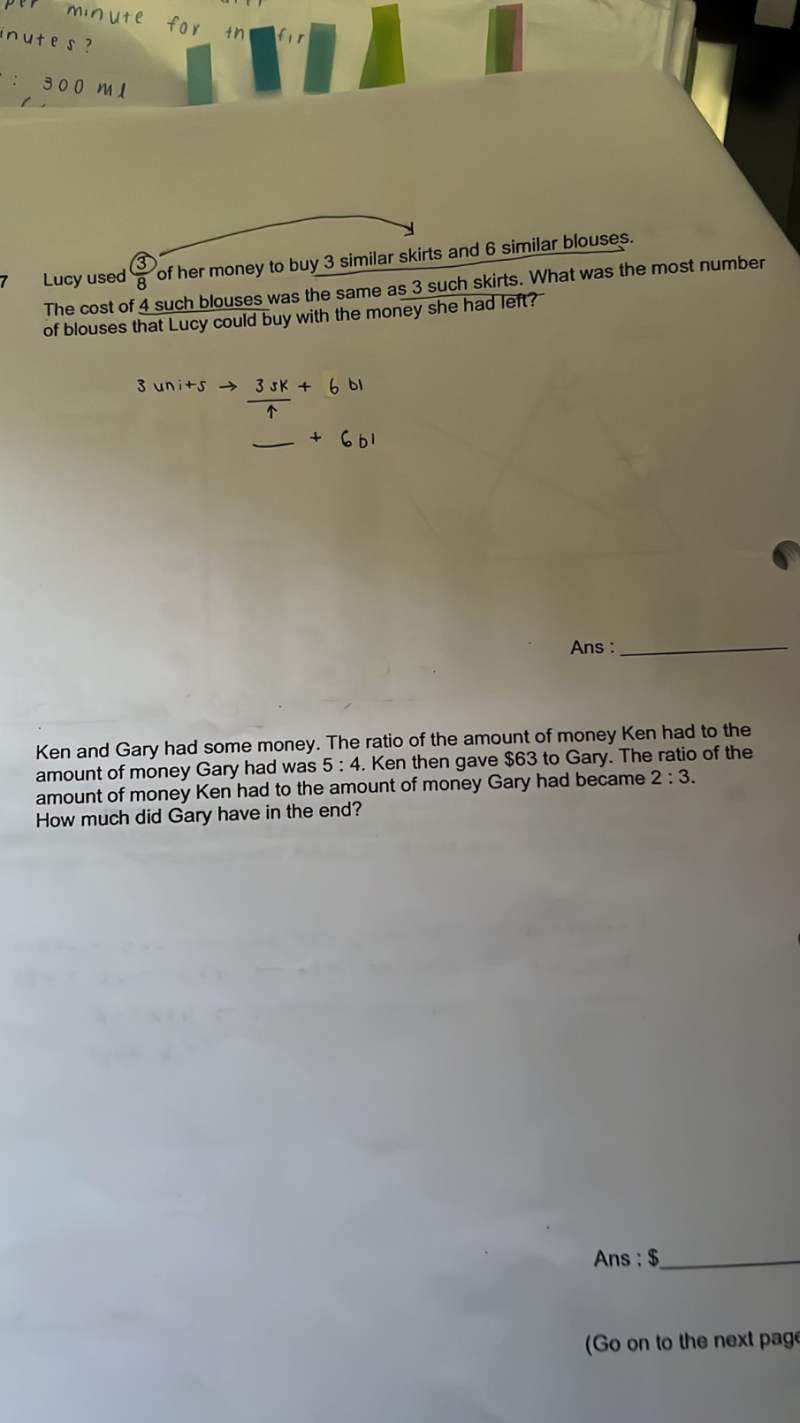# QuestionThank you

(7)  With 3units of money,   3S + 6B is bought

Given  4B = 3S  =>  4B + 6B = 10B

3units  —>  10 blouses,   remaining money = 5 units

5units   —->  10/3 x 5 =  16  23

Hence the most number of blouses that could be bought with the remaining money is 16.

(8)                        Ken                            Gary

At first              5u                                  4u

-63                              +63

In the end          2p                                  3p

5u-63=2p    ( x 3 on both sides)

15u – 189 = 6p

4u + 63 = 3p   ( x 2 on both sides)

8u + 126 = 6p

So  15u – 189  =  8u + 126

7u = 315

u = 45

4u+63 = 4(45) + 63 = 243

In the end Gary has \$243

0 Replies 1 Like